# Maharashtra Board (MSBSHSE) 2019 Question Paper 2 for Class 10th Maths In PDF

## Maharashtra SSC Maths Exam Question Paper 2019 Paper 2 with Solutions – Free Download

MSBSHSE (Maharashtra board) SSC Class 10 Maths 2019 Question Paper 2 with solutions are provided at BYJU’S. Students can download all the solutions of MSBSHSE SSC Class 10 Maths previous year board question papers in a pdf format. These solutions have detailed explanations, along with the required images and graphs, so that students can understand them easily. Practising all these SSC question papers of Maharashtra board will help the students in understanding the pattern of the exam and the type of questions that appear in the examination. This again helps in building the preparation strategy for the board exam, in such a way that students will be able to answer all the questions accurately.

### MSBSHSE Class 10 Mathematics Question Paper 2019 Paper 2 with Solutions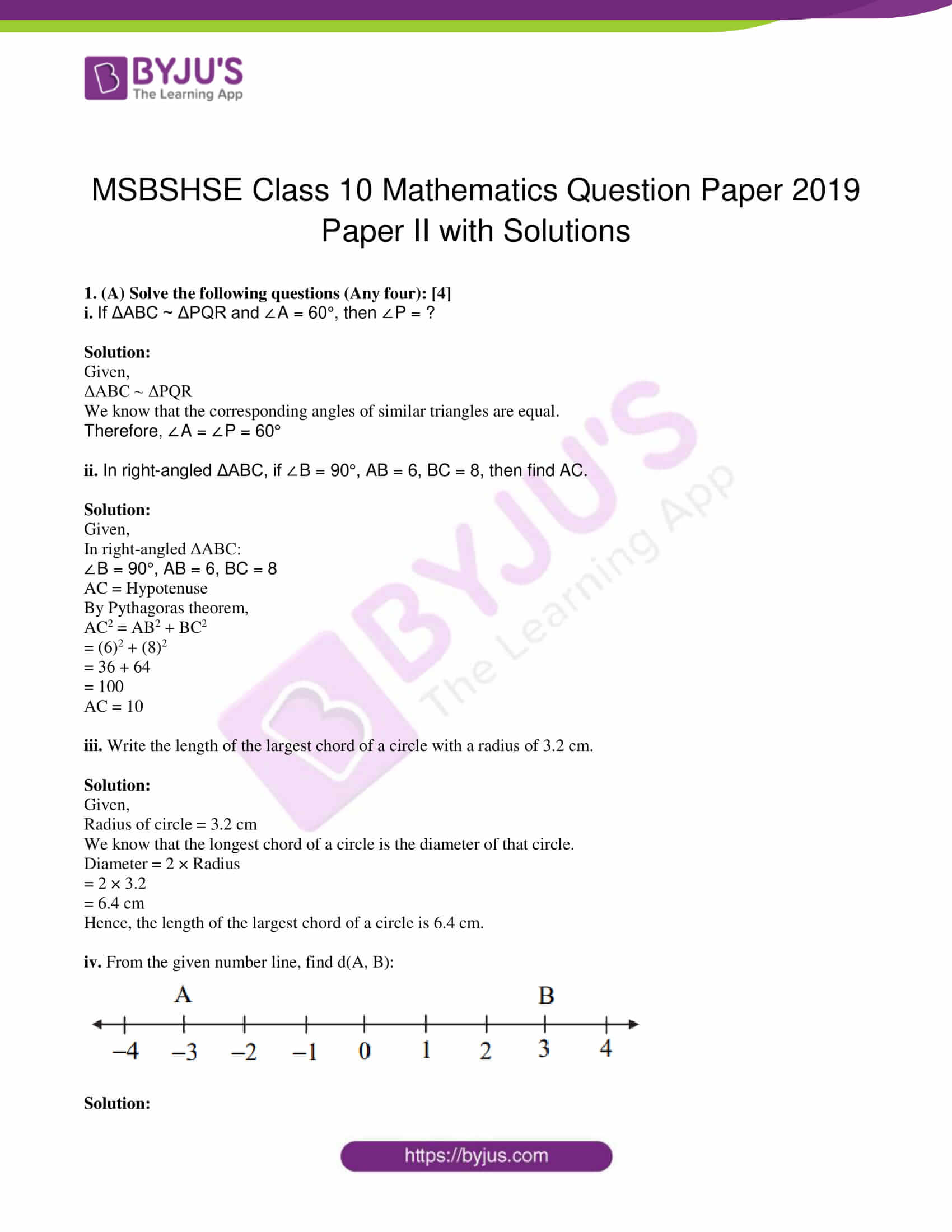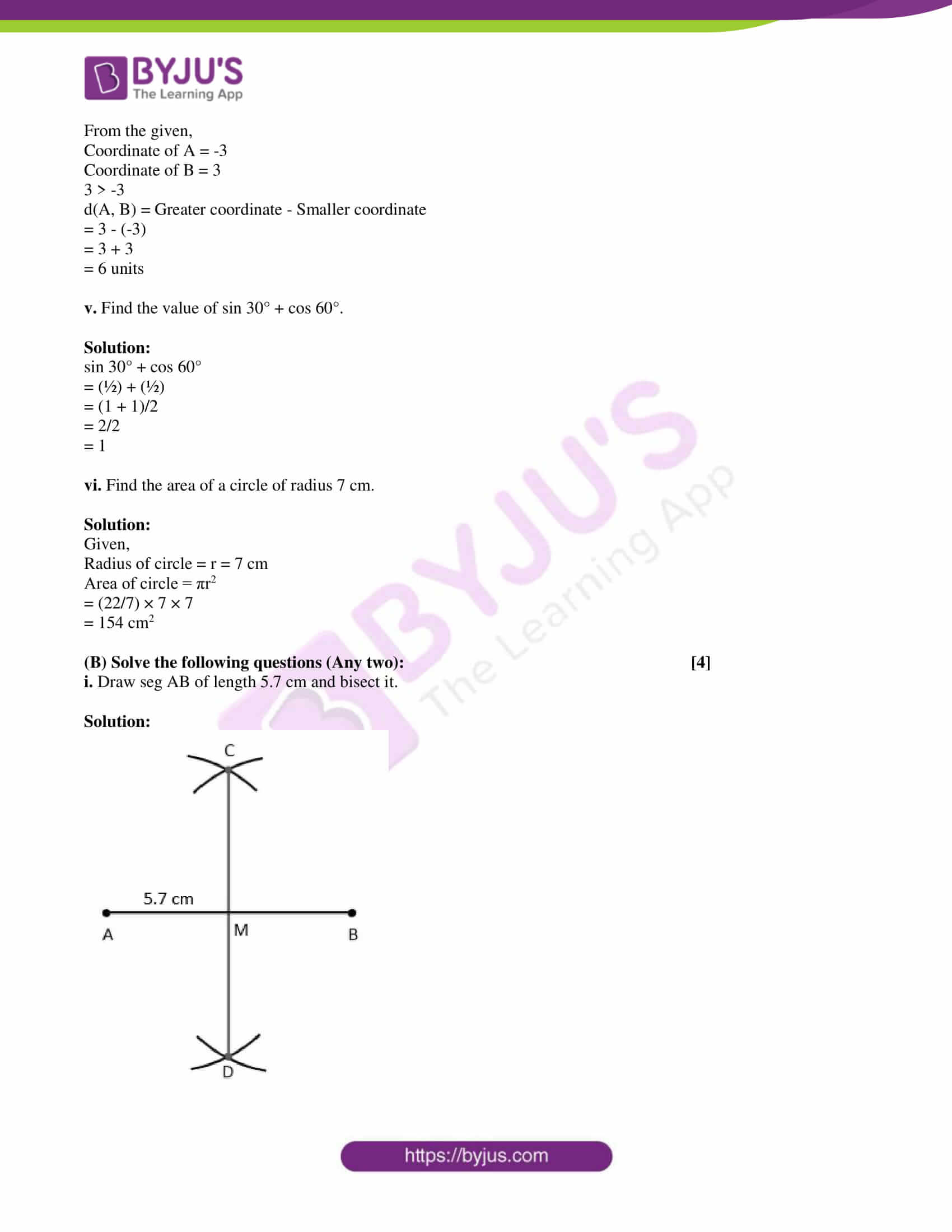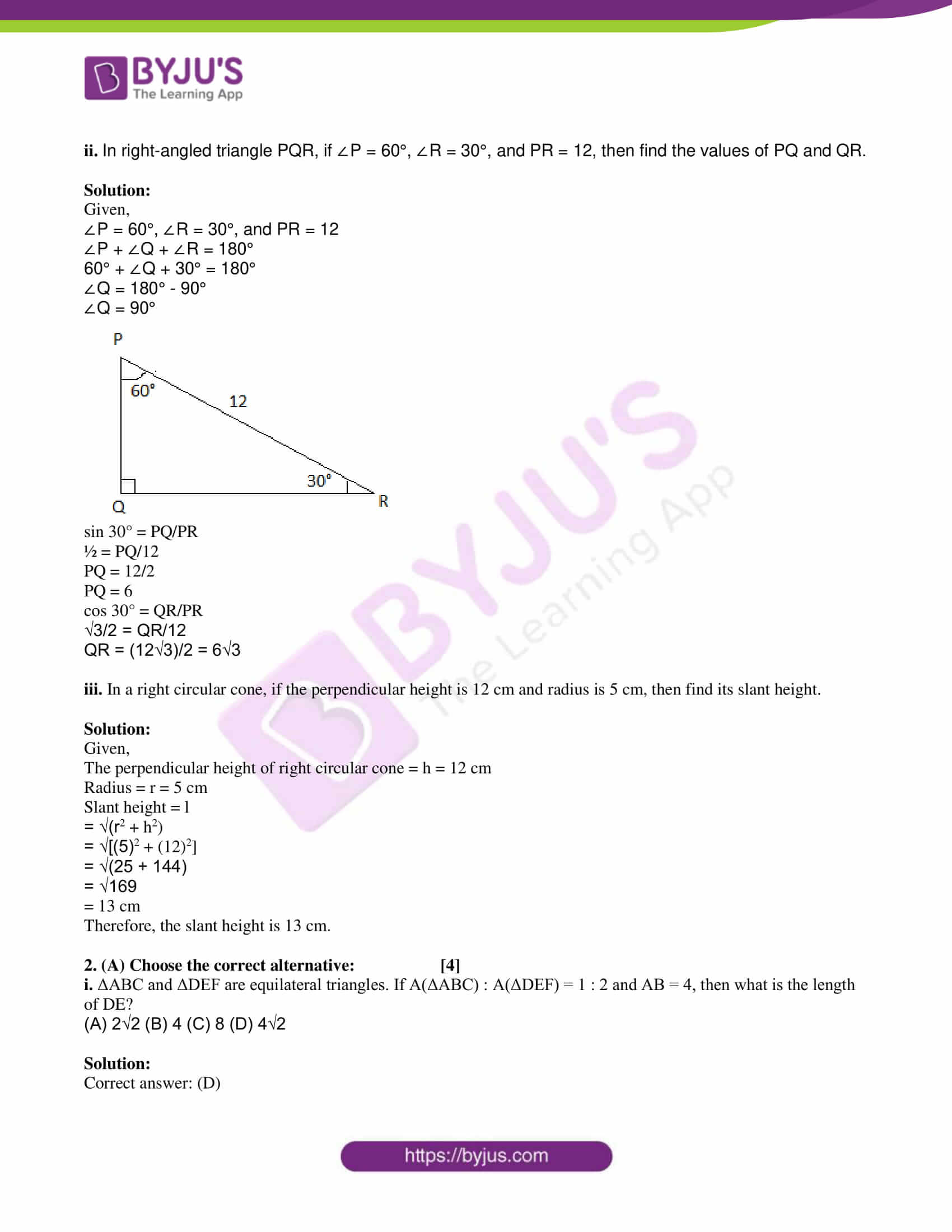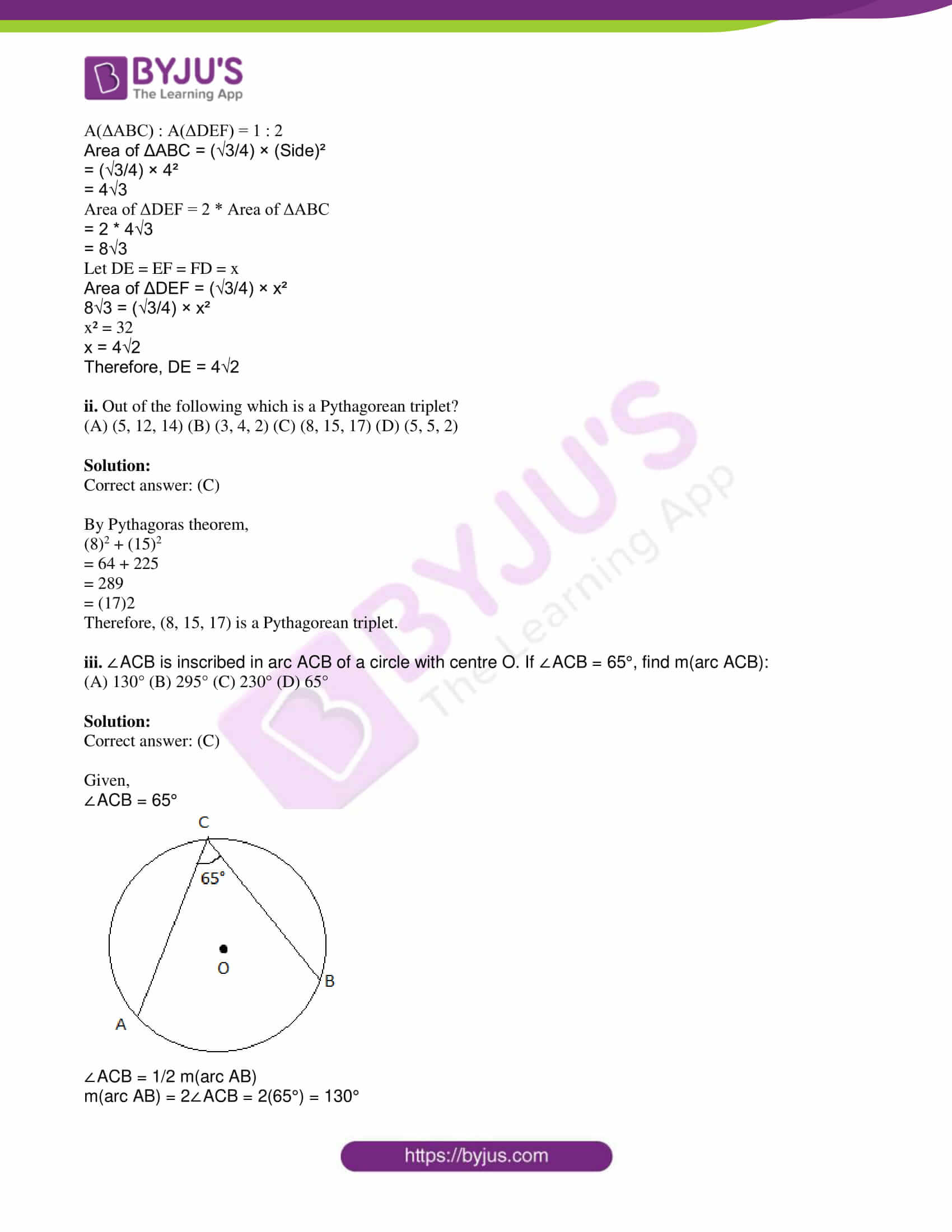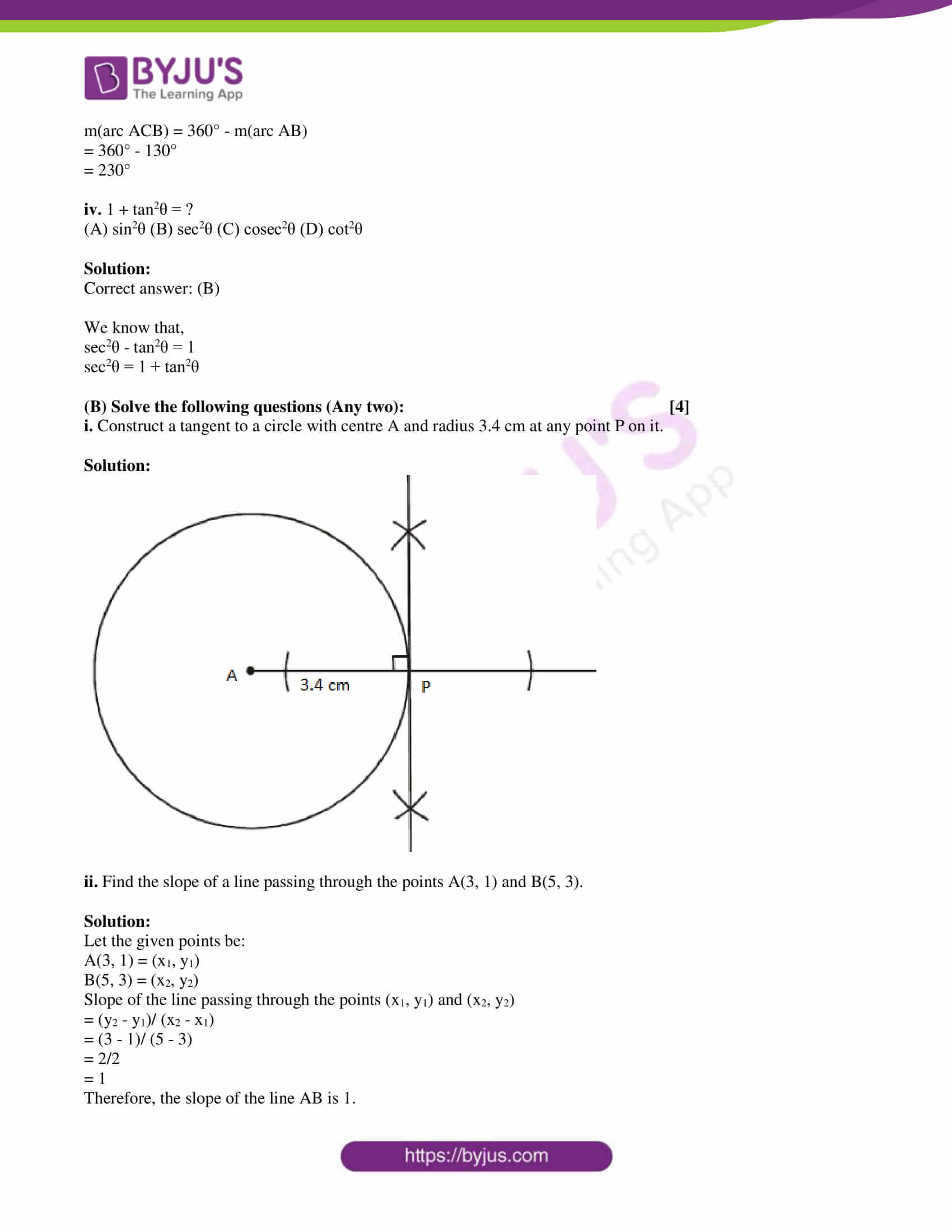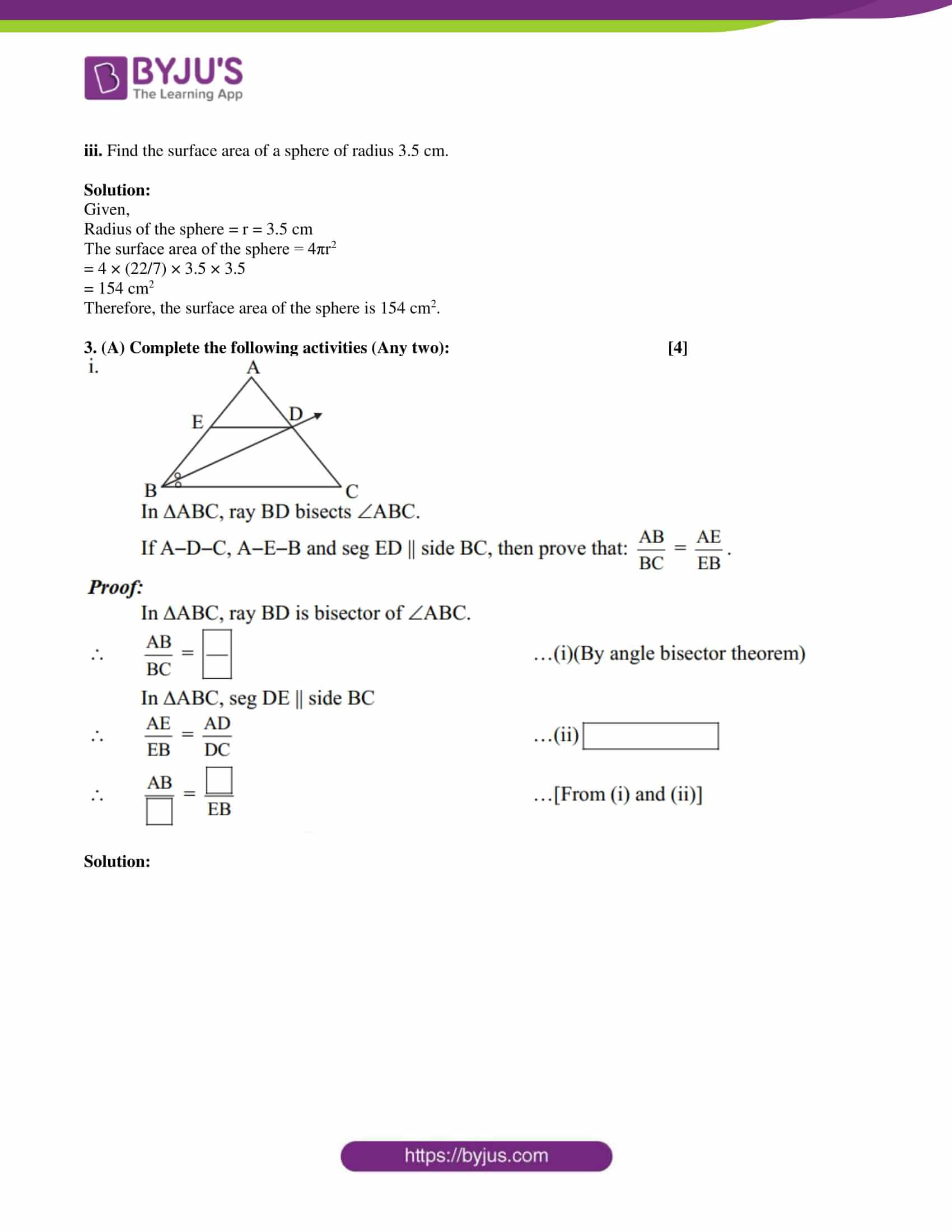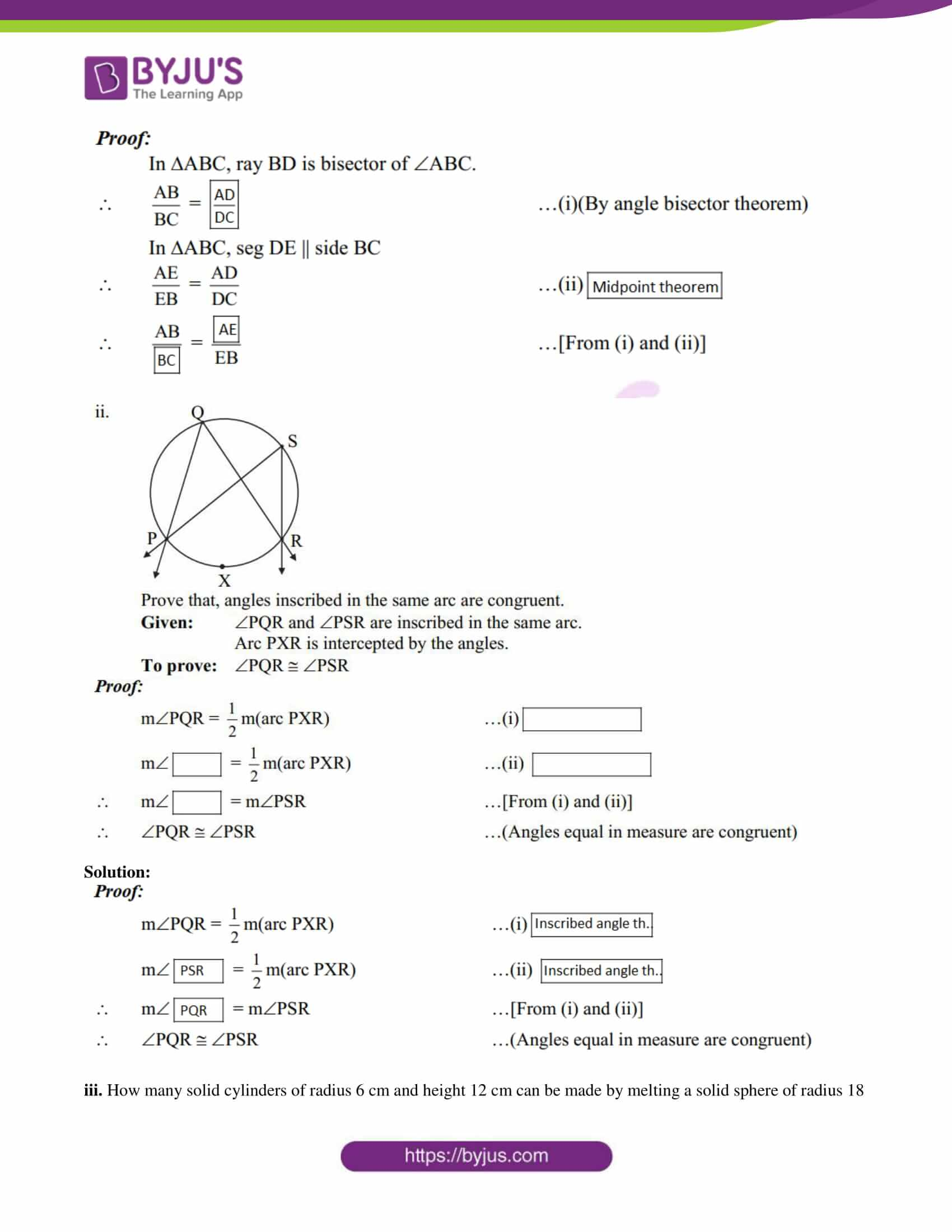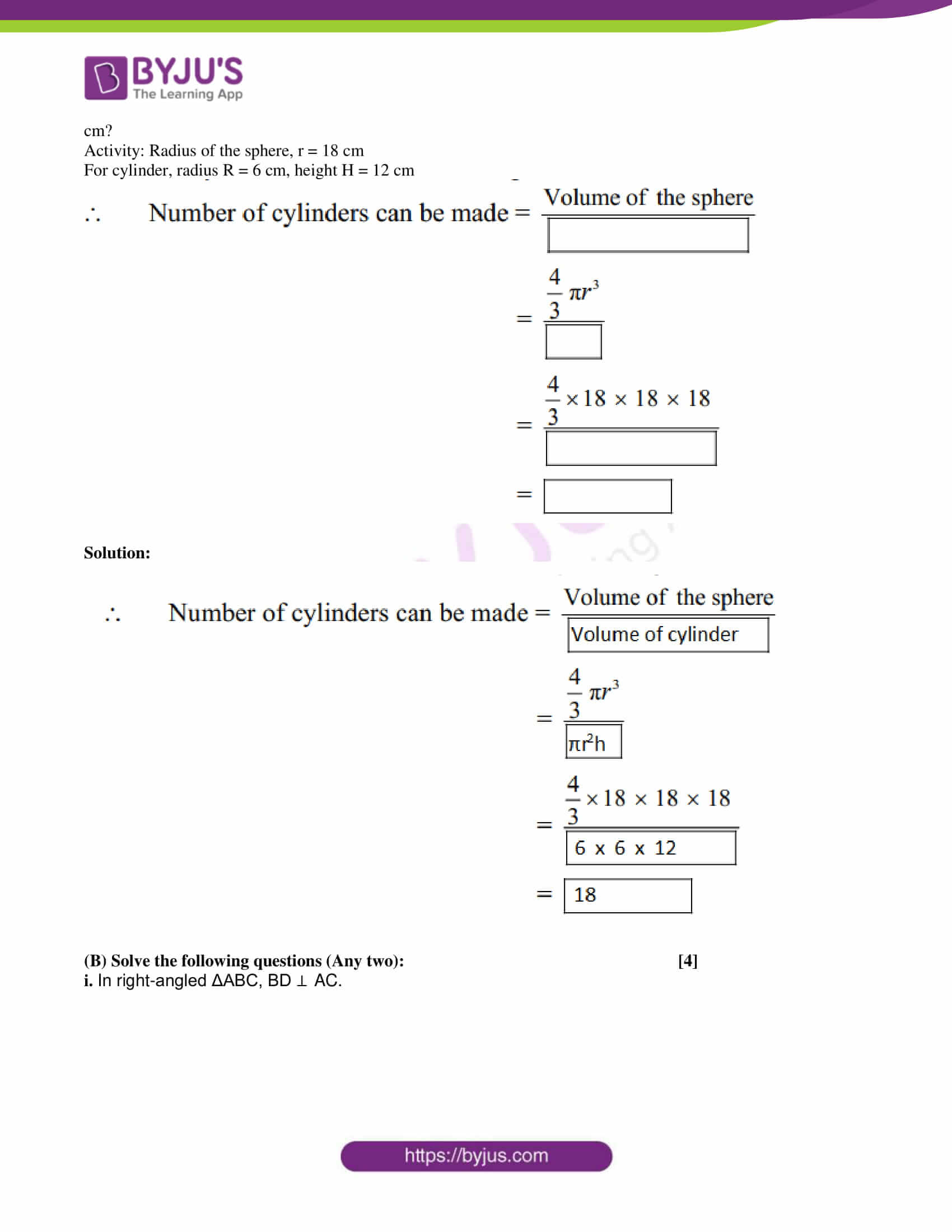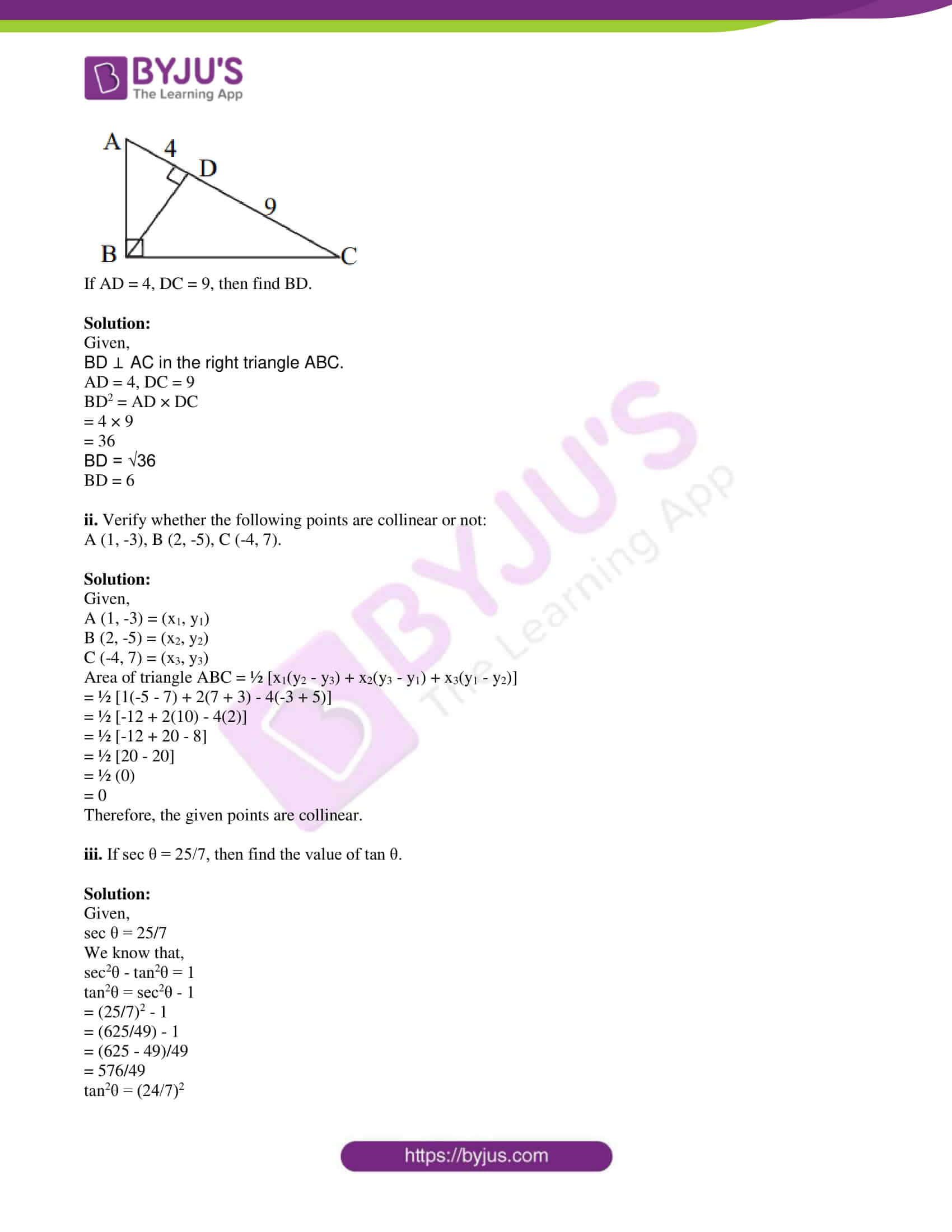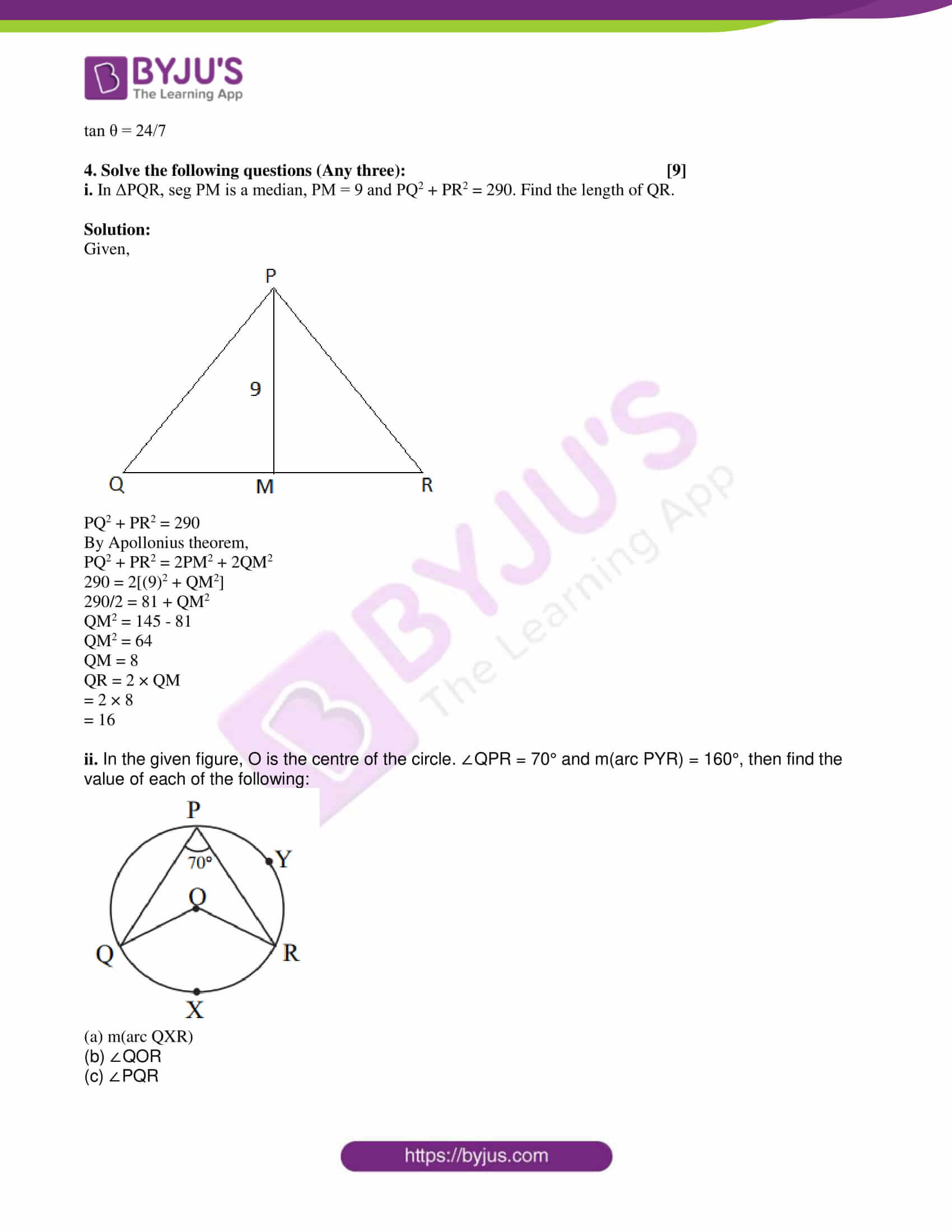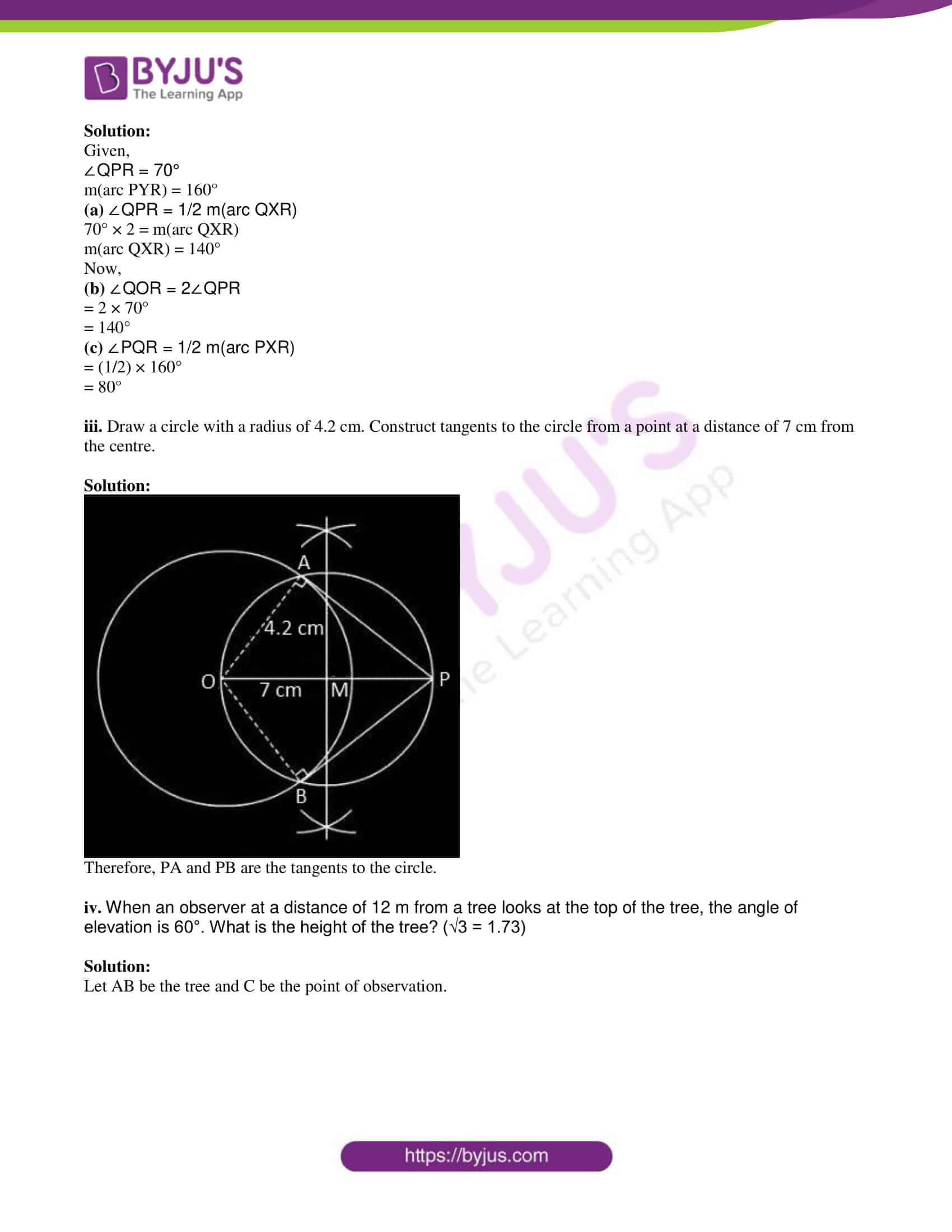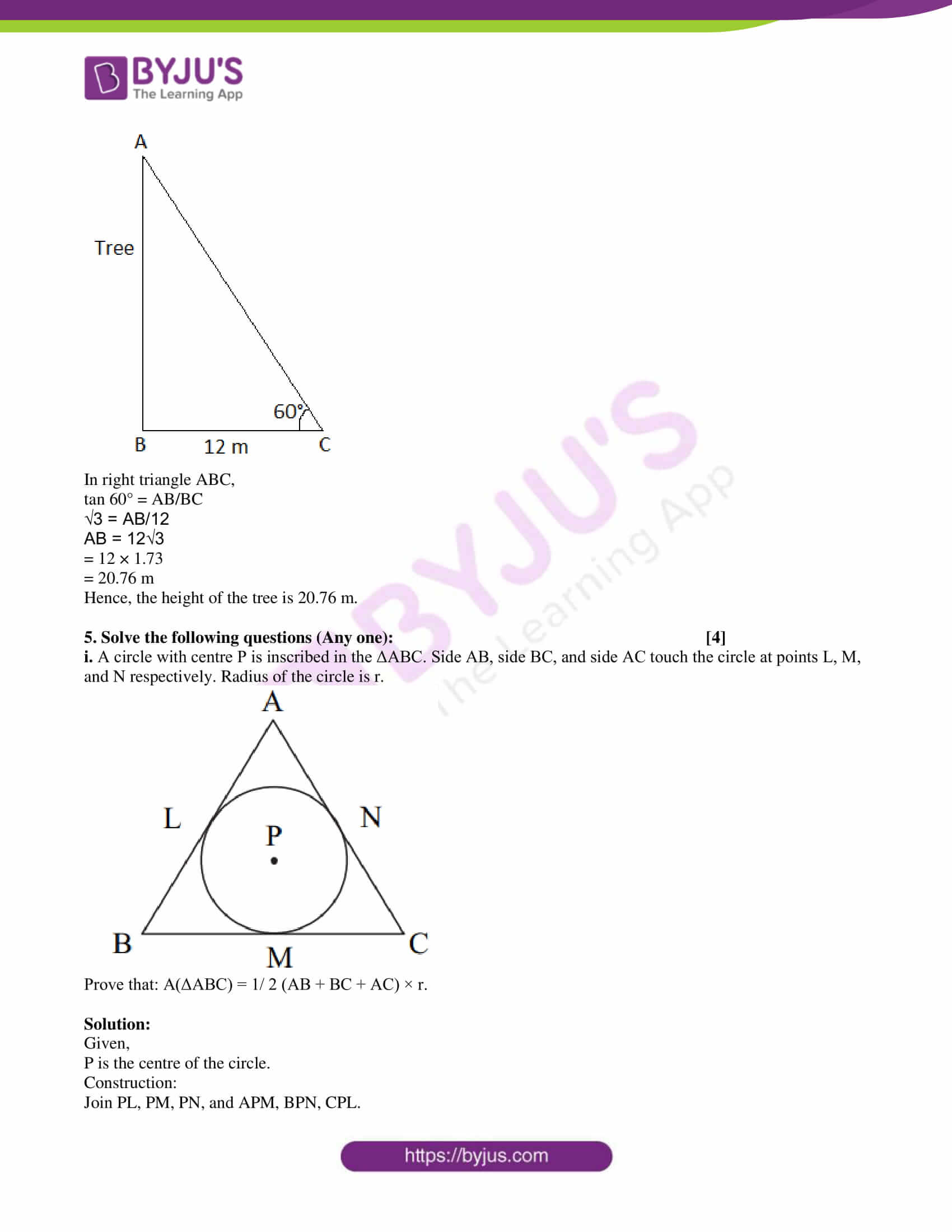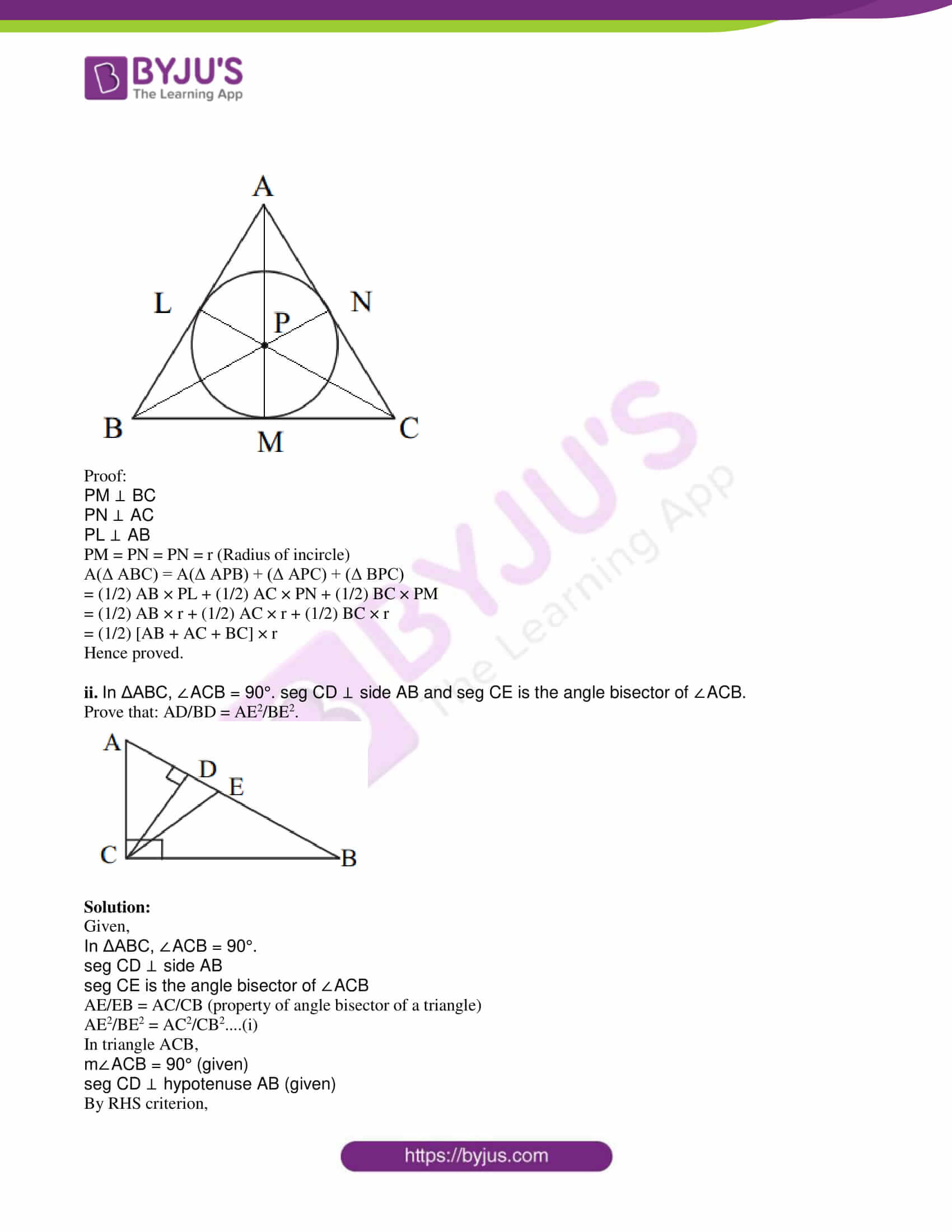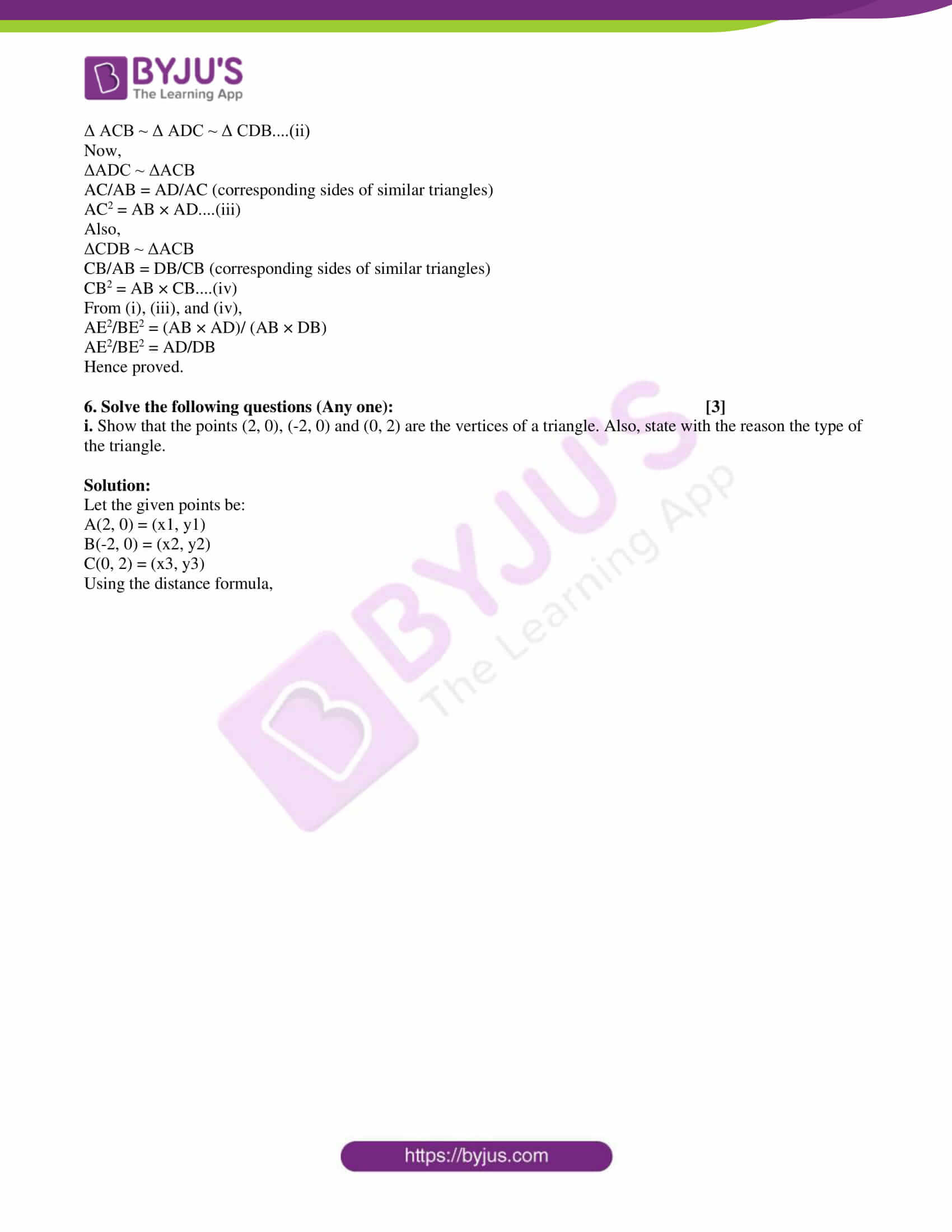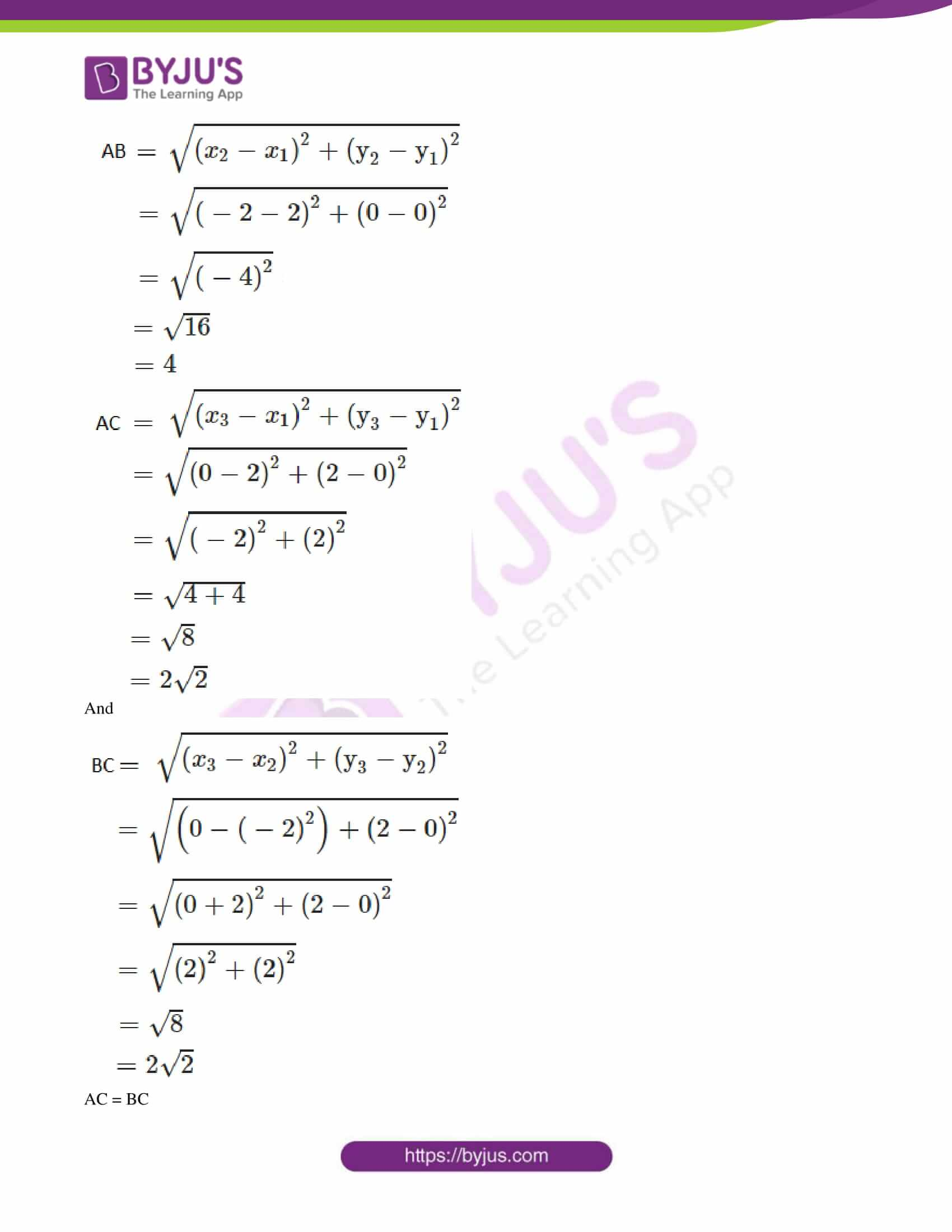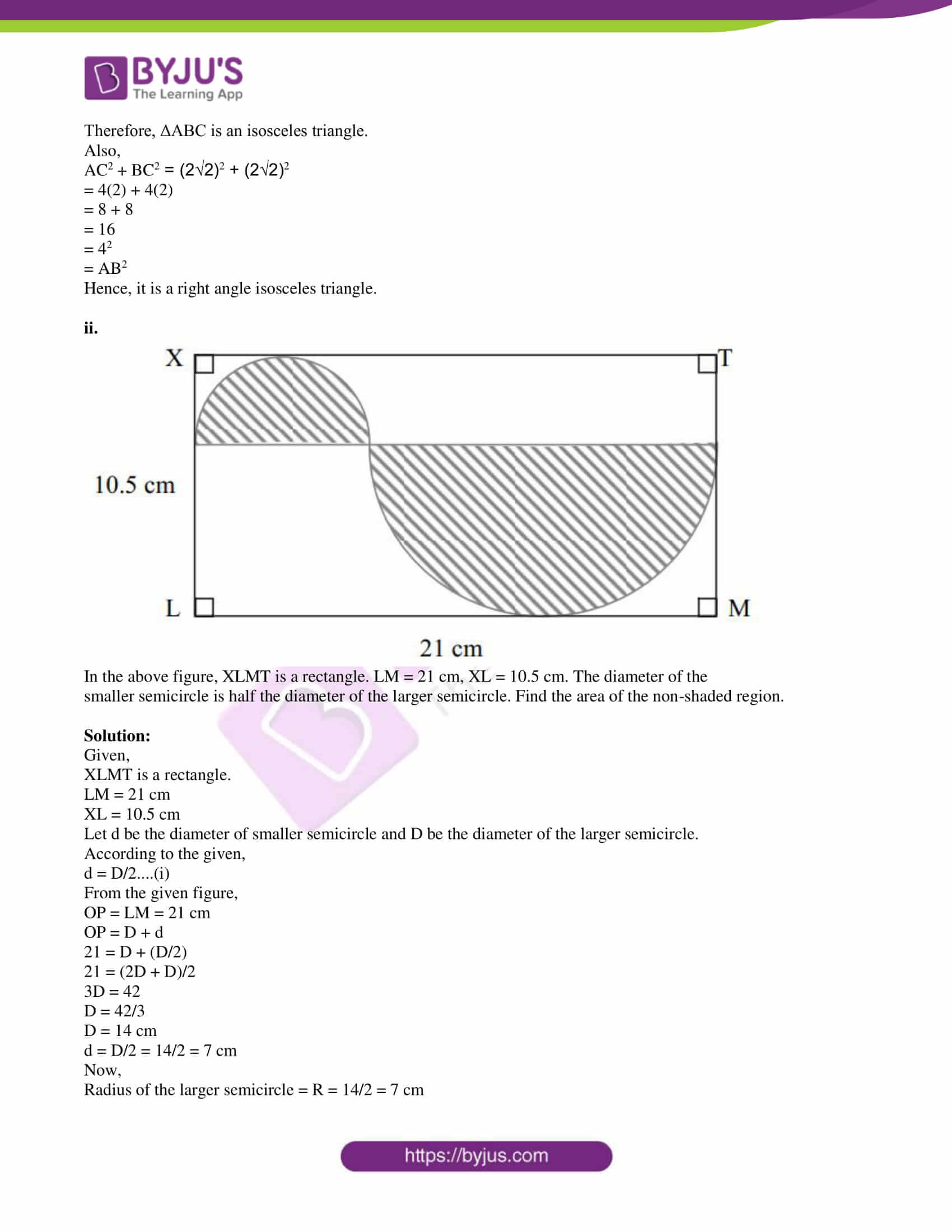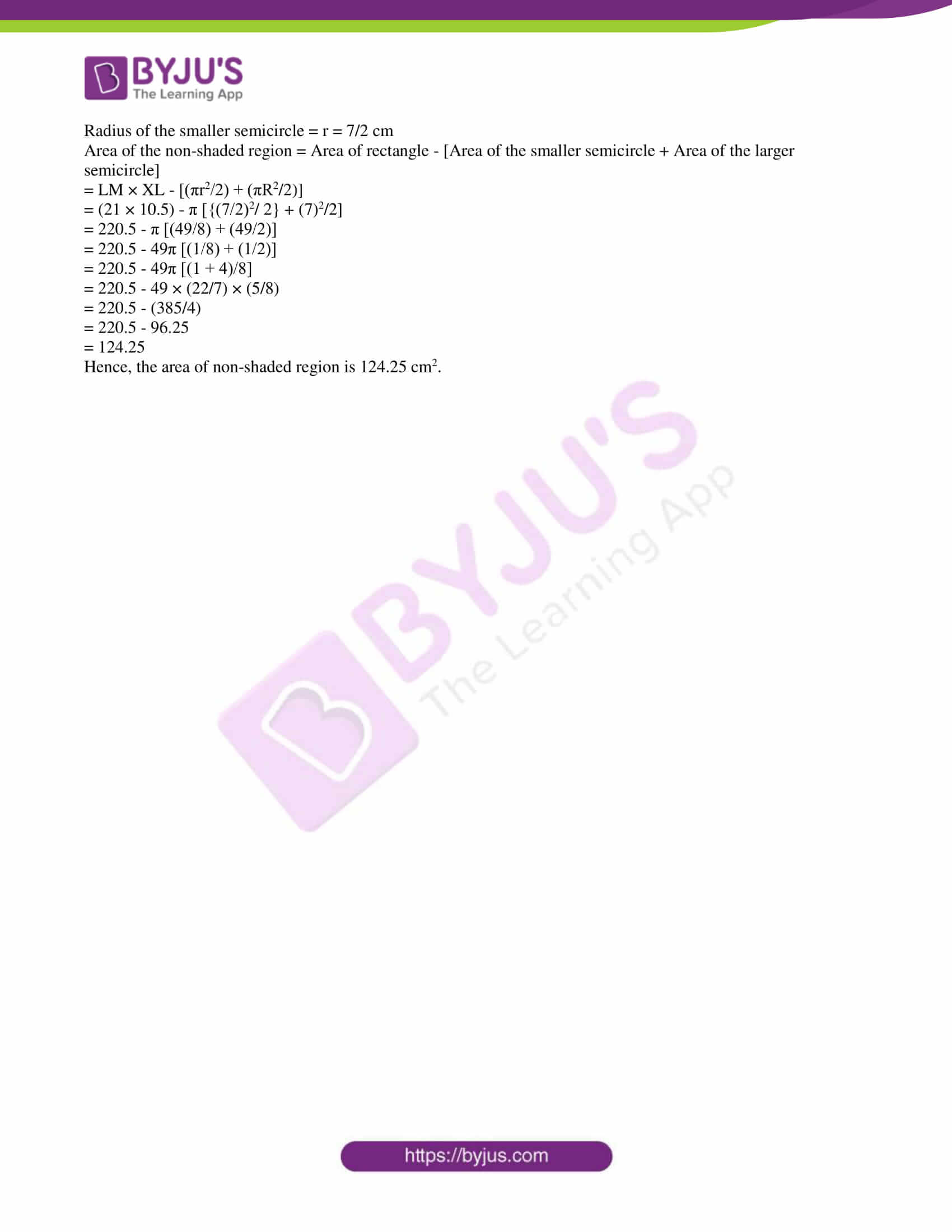1. (A) Solve the following questions (Any four): 

i. If ΔABC ~ ΔPQR and ∠A = 60°, then ∠P = ?

Solution:

Given,

ΔABC ~ ΔPQR

We know that the corresponding angles of similar triangles are equal.

Therefore, ∠A = ∠P = 60°

ii. In right-angled ΔABC, if ∠B = 90°, AB = 6, BC = 8, then find AC.

Solution:

Given,

In right-angled ΔABC:

∠B = 90°, AB = 6, BC = 8

AC = Hypotenuse

By Pythagoras theorem,

AC2 = AB2 + BC2

= (6)2 + (8)2

= 36 + 64

= 100

AC = 10

iii. Write the length of the largest chord of a circle with a radius of 3.2 cm.

Solution:

Given,

Radius of circle = 3.2 cm

We know that the longest chord of a circle is the diameter of that circle.

= 2 × 3.2

= 6.4 cm

Hence, the length of the largest chord of a circle is 6.4 cm.

iv. From the given number line, find d(A, B):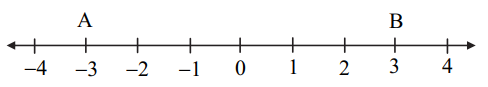Solution:

From the given,

Coordinate of A = -3

Coordinate of B = 3

3 > -3

d(A, B) = Greater coordinate – Smaller coordinate

= 3 – (-3)

= 3 + 3

= 6 units

v. Find the value of sin 30° + cos 60°.

Solution:

sin 30° + cos 60°

= (½) + (½)

= (1 + 1)/2

= 2/2

= 1

vi. Find the area of a circle of radius 7 cm.

Solution:

Given,

Radius of circle = r = 7 cm

Area of circle = πr2

= (22/7) × 7 × 7

= 154 cm2

(B) Solve the following questions (Any two): 

i. Draw seg AB of length 5.7 cm and bisect it.

Solution: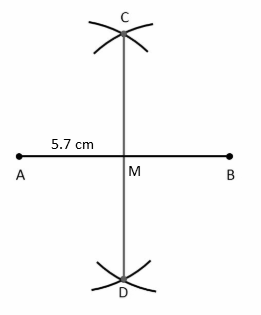ii. In right-angled triangle PQR, if ∠P = 60°, ∠R = 30°, and PR = 12, then find the values of PQ and QR.

Solution:

Given,

∠P = 60°, ∠R = 30°, and PR = 12

∠P + ∠Q + ∠R = 180°

60° + ∠Q + 30° = 180°

∠Q = 180° – 90°

∠Q = 90°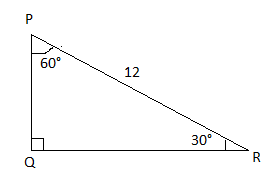sin 30° = PQ/PR

½ = PQ/12

PQ = 12/2

PQ = 6

cos 30° = QR/PR

√3/2 = QR/12

QR = (12√3)/2 = 6√3

iii. In a right circular cone, if the perpendicular height is 12 cm and radius is 5 cm, then find its slant height.

Solution:

Given,

The perpendicular height of right circular cone = h = 12 cm

Radius = r = 5 cm

Slant height = l

= √(r2 + h2)

= √[(5)2 + (12)2]

= √(25 + 144)

= √169

= 13 cm

Therefore, the slant height is 13 cm.

2. (A) Choose the correct alternative: 

i. ΔABC and ΔDEF are equilateral triangles. If A(ΔABC) : A(ΔDEF) = 1 : 2 and AB = 4, then what is the length of DE?

(A) 2√2 (B) 4 (C) 8 (D) 4√2

Solution:

A(ΔABC) : A(ΔDEF) = 1 : 2

Area of ΔABC = (√3/4) × (Side)²

= (√3/4) × 4²

= 4√3

Area of ΔDEF = 2 * Area of ΔABC

= 2 * 4√3

= 8√3

Let DE = EF = FD = x

Area of ΔDEF = (√3/4) × x²

8√3 = (√3/4) × x²

x² = 32

x = 4√2

Therefore, DE = 4√2

ii. Out of the following which is a Pythagorean triplet?

(A) (5, 12, 14) (B) (3, 4, 2) (C) (8, 15, 17) (D) (5, 5, 2)

Solution:

By Pythagoras theorem,

(8)2 + (15)2

= 64 + 225

= 289

= (17)2

Therefore, (8, 15, 17) is a Pythagorean triplet.

iii. ∠ACB is inscribed in arc ACB of a circle with centre O. If ∠ACB = 65°, find m(arc ACB):

(A) 130° (B) 295° (C) 230° (D) 65°

Solution:

Given,

∠ACB = 65°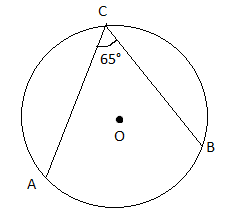∠ACB = 1/2 m(arc AB)

m(arc AB) = 2∠ACB = 2(65°) = 130°

m(arc ACB) = 360° – m(arc AB)

= 360° – 130°

= 230°

iv. 1 + tan2θ = ?

(A) sin2θ (B) sec2θ (C) cosec2θ (D) cot2θ

Solution:

We know that,

sec2θ – tan2θ = 1

sec2θ = 1 + tan2θ

(B) Solve the following questions (Any two): 

i. Construct a tangent to a circle with centre A and radius 3.4 cm at any point P on it.

Solution: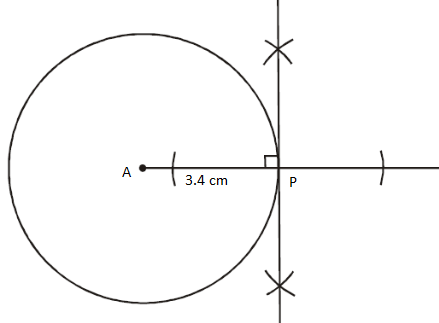ii. Find the slope of a line passing through the points A(3, 1) and B(5, 3).

Solution:

Let the given points be:

A(3, 1) = (x1, y1)

B(5, 3) = (x2, y2)

Slope of the line passing through the points (x1, y1) and (x2, y2)

= (y2 – y1)/ (x2 – x1)

= (3 – 1)/ (5 – 3)

= 2/2

= 1

Therefore, the slope of the line AB is 1.

iii. Find the surface area of a sphere of radius 3.5 cm.

Solution:

Given,

Radius of the sphere = r = 3.5 cm

The surface area of the sphere = 4πr2

= 4 × (22/7) × 3.5 × 3.5

= 154 cm2

Therefore, the surface area of the sphere is 154 cm2.

3. (A) Complete the following activities (Any two):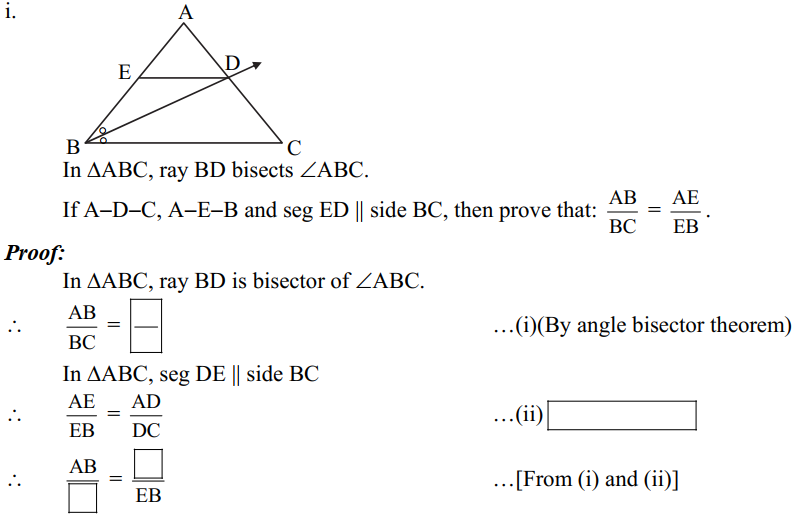Solution: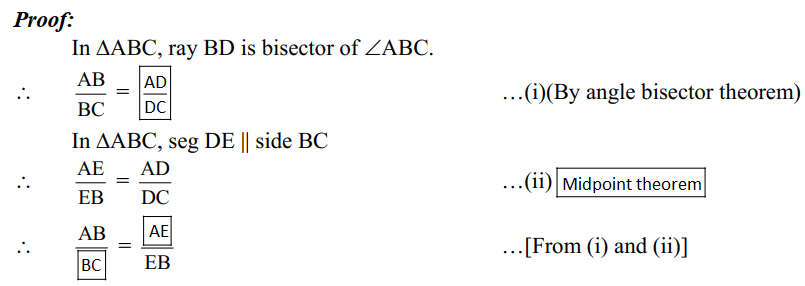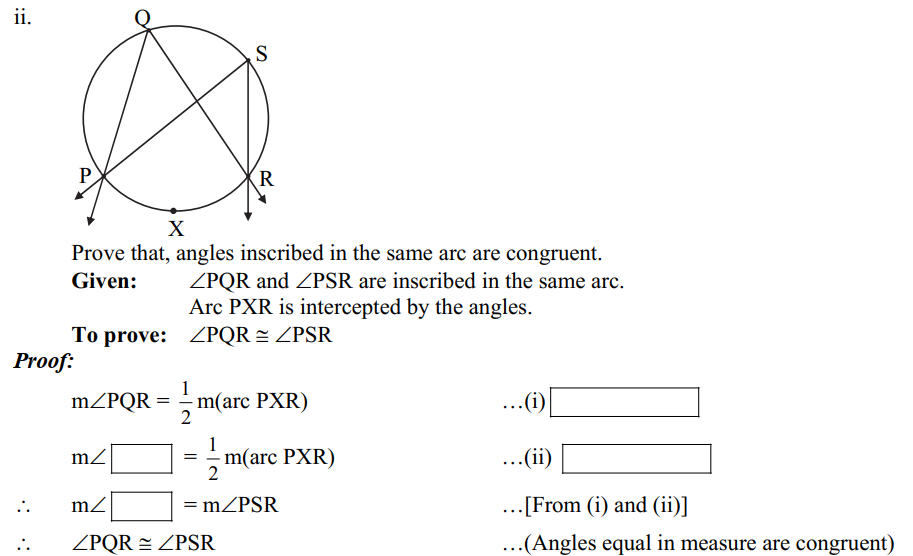Solution: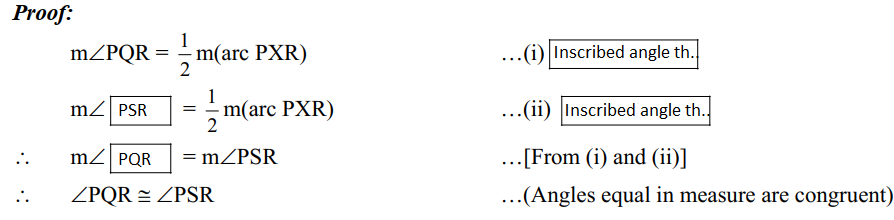iii. How many solid cylinders of radius 6 cm and height 12 cm can be made by melting a solid sphere of radius 18 cm?

Activity: Radius of the sphere, r = 18 cm

For cylinder, radius R = 6 cm, height H = 12 cm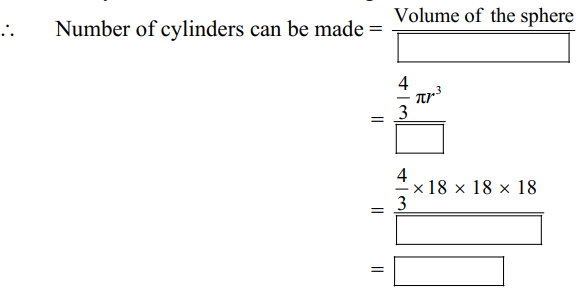Solution: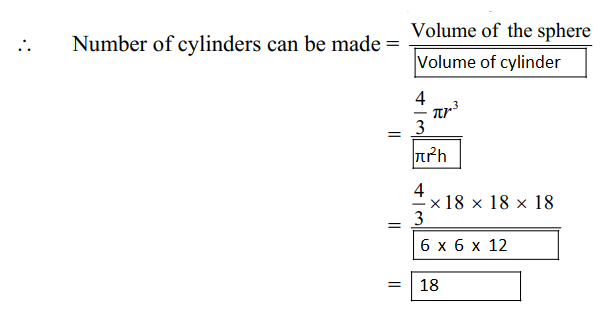(B) Solve the following questions (Any two): 

i. In right-angled ΔABC, BD ⊥ AC.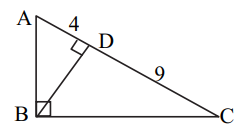If AD = 4, DC = 9, then find BD.

Solution:

Given,

BD ⊥ AC in the right triangle ABC.

AD = 4, DC = 9

= 4 × 9

= 36

BD = √36

BD = 6

ii. Verify whether the following points are collinear or not:

A (1, -3), B (2, -5), C (-4, 7).

Solution:

Given,

A (1, -3) = (x1, y1)

B (2, -5) = (x2, y2)

C (-4, 7) = (x3, y3)

Area of triangle ABC = ½ [x1(y2 – y3) + x2(y3 – y1) + x3(y1 – y2)]

= ½ [1(-5 – 7) + 2(7 + 3) – 4(-3 + 5)]

= ½ [-12 + 2(10) – 4(2)]

= ½ [-12 + 20 – 8]

= ½ [20 – 20]

= ½ (0)

= 0

Therefore, the given points are collinear.

iii. If sec θ = 25/7, then find the value of tan θ.

Solution:

Given,

sec θ = 25/7

We know that,

sec2θ – tan2θ = 1

tan2θ = sec2θ – 1

= (25/7)2 – 1

= (625/49) – 1

= (625 – 49)/49

= 576/49

4. Solve the following questions (Any three): 

i. In ΔPQR, seg PM is a median, PM = 9 and PQ2 + PR2 = 290. Find the length of QR.

Solution:

Given,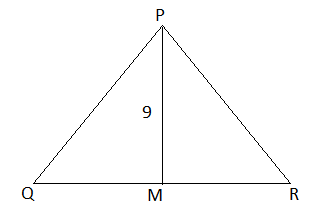PQ2 + PR2 = 290

By Apollonius theorem,

PQ2 + PR2 = 2PM2 + 2QM2

290 = 2[(9)2 + QM2]

290/2 = 81 + QM2

QM2 = 145 – 81

QM2 = 64

QM = 8

QR = 2 × QM

= 2 × 8

= 16

ii. In the given figure, O is the centre of the circle. ∠QPR = 70° and m(arc PYR) = 160°, then find the value of each of the following: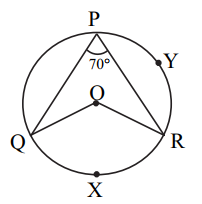(a) m(arc QXR)

(b) ∠QOR

(c) ∠PQR

Solution:

Given,

∠QPR = 70°

m(arc PYR) = 160°

(a) ∠QPR = 1/2 m(arc QXR)

70° × 2 = m(arc QXR)

m(arc QXR) = 140°

Now,

(b) ∠QOR = 2∠QPR

= 2 × 70°

= 140°

(c) ∠PQR = 1/2 m(arc PXR)

= (1/2) × 160°

= 80°

iii. Draw a circle with a radius of 4.2 cm. Construct tangents to the circle from a point at a distance of 7 cm from the centre.

Solution: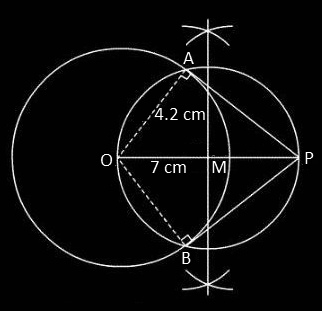Therefore, PA and PB are the tangents to the circle.

iv. When an observer at a distance of 12 m from a tree looks at the top of the tree, the angle of elevation is 60°. What is the height of the tree? (√3 = 1.73)

Solution:

Let AB be the tree and C be the point of observation.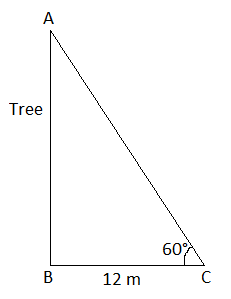In right triangle ABC,

tan 60° = AB/BC

√3 = AB/12

AB = 12√3

= 12 × 1.73

= 20.76 m

Hence, the height of the tree is 20.76 m.

5. Solve the following questions (Any one): 

i. A circle with centre P is inscribed in the ΔABC. Side AB, side BC, and side AC touch the circle at points L, M, and N respectively. Radius of the circle is r.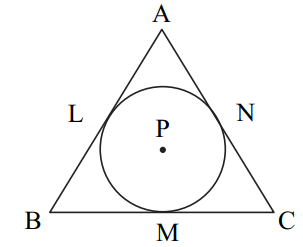Prove that: A(ΔABC) = 1/ 2 (AB + BC + AC) × r.

Solution:

Given,

P is the centre of the circle.

Construction:

Join PL, PM, PN, and APM, BPN, CPL.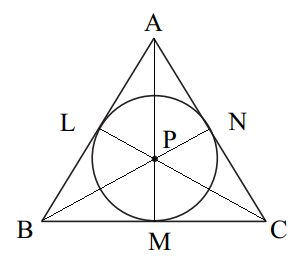Proof:

PM ⊥ BC

PN ⊥ AC

PL ⊥ AB

PM = PN = PN = r (Radius of incircle)

A(Δ ABC) = A(Δ APB) + (Δ APC) + (Δ BPC)

= (1/2) AB × PL + (1/2) AC × PN + (1/2) BC × PM

= (1/2) AB × r + (1/2) AC × r + (1/2) BC × r

= (1/2) [AB + AC + BC] × r

Hence proved.

ii. In ΔABC, ∠ACB = 90°. seg CD ⊥ side AB and seg CE is the angle bisector of ∠ACB.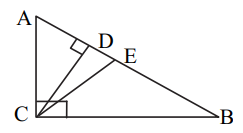Solution:

Given,

In ΔABC, ∠ACB = 90°.

seg CD ⊥ side AB

seg CE is the angle bisector of ∠ACB

AE/EB = AC/CB (property of angle bisector of a triangle)

AE2/BE2 = AC2/CB2….(i)

In triangle ACB,

m∠ACB = 90° (given)

seg CD ⊥ hypotenuse AB (given)

By RHS criterion,

Δ ACB ~ Δ ADC ~ Δ CDB….(ii)

Now,

AC/AB = AD/AC (corresponding sides of similar triangles)

Also,

ΔCDB ~ ΔACB

CB/AB = DB/CB (corresponding sides of similar triangles)

CB2 = AB × CB….(iv)

From (i), (iii), and (iv),

AE2/BE2 = (AB × AD)/ (AB × DB)

Hence proved.

6. Solve the following questions (Any one): 

i. Show that the points (2, 0), (-2, 0) and (0, 2) are the vertices of a triangle. Also, state with the reason the type of the triangle.

Solution:

Let the given points be:

A(2, 0) = (x1, y1)

B(-2, 0) = (x2, y2)

C(0, 2) = (x3, y3)

Using the distance formula,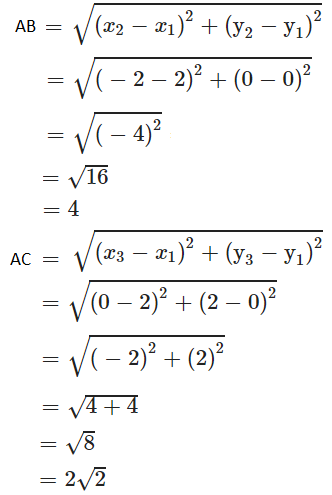And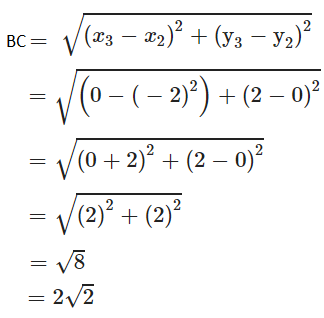AC = BC

Therefore, ΔABC is an isosceles triangle.

Also,

AC2 + BC2 = (2√2)2 + (2√2)2

= 4(2) + 4(2)

= 8 + 8

= 16

= 42

= AB2

Hence, it is a right angle isosceles triangle.

ii.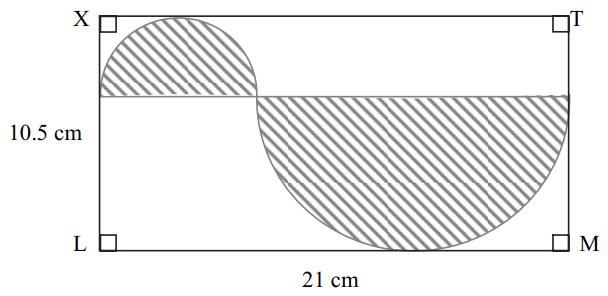In the above figure, XLMT is a rectangle. LM = 21 cm, XL = 10.5 cm. The diameter of the

smaller semicircle is half the diameter of the larger semicircle. Find the area of the non-shaded region.

Solution:

Given,

XLMT is a rectangle.

LM = 21 cm

XL = 10.5 cm

Let d be the diameter of the smaller semicircle and D be the diameter of the larger semicircle.

According to the given,

d = D/2….(i)

From the given figure,

OP = LM = 21 cm

OP = D + d

21 = D + (D/2)

21 = (2D + D)/2

3D = 42

D = 42/3

D = 14 cm

d = D/2 = 14/2 = 7 cm

Now,

Radius of the larger semicircle = R = 14/2 = 7 cm

Radius of the smaller semicircle = r = 7/2 cm

Area of the non-shaded region = Area of rectangle – [Area of the smaller semicircle + Area of the larger semicircle]

= LM × XL – [(πr2/2) + (πR2/2)]

= (21 × 10.5) – π [{(7/2)2/ 2} + (7)2/2]

= 220.5 – π [(49/8) + (49/2)]

= 220.5 – 49π [(1/8) + (1/2)]

= 220.5 – 49π [(1 + 4)/8]

= 220.5 – 49 × (22/7) × (5/8)

= 220.5 – (385/4)

= 220.5 – 96.25

= 124.25

Hence, the area of the non-shaded region is 124.25 cm2.